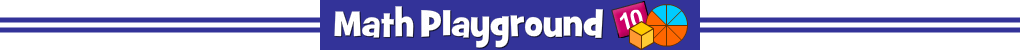Space Race Multiplication
NUMBER OF PLAYERS: 4
The space racers are lined up and ready to go.
Practice multiplication facts and win this year's galaxy games.
Space Race Multiplication - Learning Connections
Essential Skills
Mental Math - multiply numbers by memory or through other efficient methods
Problem Solving - match multiplication expressions with the correct products

Common Core Connection for 3rd Grade
Determine the unknown whole number in a multiplication equation (4 x 6 = ?).
Apply properties of operations as strategies to multiply.